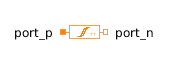Complex Step - MapleSim Help

Complex Step

Generate step signal of type ComplexDescription The Complex Step component outputs a complex step.Equations $y=\mathrm{offset}+\left\{\begin{array}{cc}0& t<{T}_{0}\\ \mathrm{height}& \mathrm{otherwise}\end{array}$Connections

 Name Description Modelica ID $y$ Complex output signal yParameters

 Name Default Units Description Modelica ID $\mathrm{height}$ $I$ Height of step height $\mathrm{offset}$ $0$ Offset of output signal y offset ${T}_{0}$ $0$ $s$ Output y = offset for time < startTime startTimeModelica Standard Library The component described in this topic is from the Modelica Standard Library. To view the original documentation, which includes author and copyright information, click here.